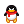# charcode是八个unsignedshort类型的数组``````count=0;for(j_v=0;j_vcsize;j_v++){unsignedshortcode,fact,insize;code=charcode[j_v];fact=n;insize=num;intii=((code*fact)%insize);index_s[ii]++;count++;}if(count!=csize){count=1;}//addupcount=0;for(intk=0;knum;k++){for(intip=0;ip20;ip++){if(index_s[k]==ip){p[ip]++;break;}}count+=index_s[k];}if(count!=csize){count=1;//debug1}count=0;for(intkk=1;kk20;kk++){count+=p[kk];}if(countcsize){count=1;}
``````